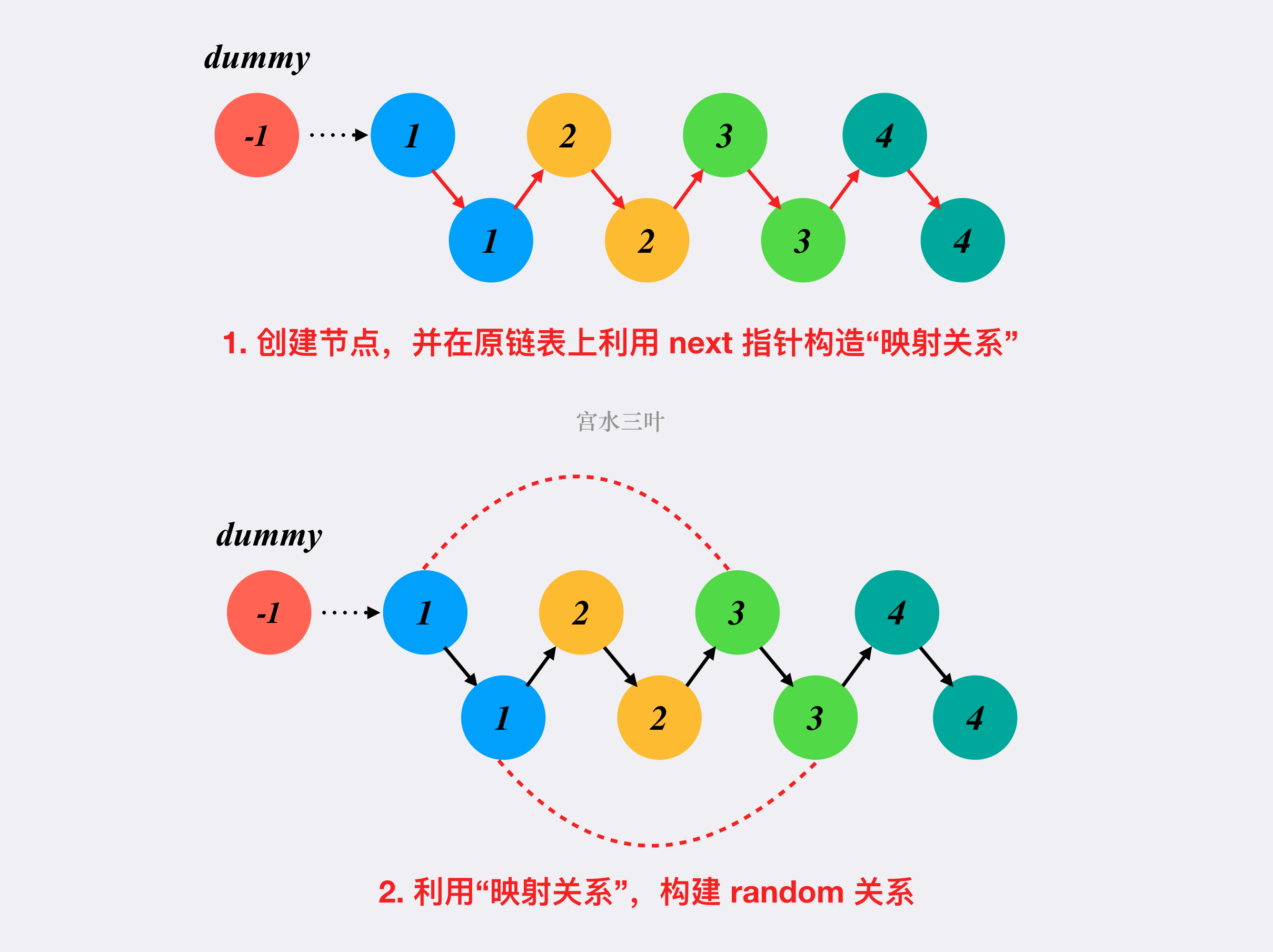# 【每日算法】复制带随机指针的链表：「哈希表」&「原地算法」｜Python 主题月

### 题目描述

Tag : 「哈希表」、「链表」

• val：一个表示 Node.val 的整数。
• random_index：随机指针指向的节点索引（范围从 0 到 n-1）；如果不指向任何节点，则为  null 。

输入：head = [[7,null],[13,0],[11,4],[10,2],[1,0]]

输入：head = [[1,1],[2,1]]

输入：head = [[3,null],[3,0],[3,null]]

输入：head = []

• 0 <= n <= 1000
• -10000 <= Node.val <= 10000

### 模拟 + 哈希表

1. 先不考虑 random 指针，和原本的链表复制一样，创建新新节点，并构造 next 指针关系，同时使用「哈希表」记录原节点和新节点的映射关系；
2. 对原链表和新链表进行同时遍历，对于原链表的每个节点上的 random 都通过「哈希表」找到对应的新 random 节点，并在新链表上构造 random 关系。

Java 代码：

class Solution {
Map<Node, Node> map = new HashMap<>();
Node dummy = new Node(-1);
Node tail = dummy, tmp = head;
while (tmp != null) {
Node node = new Node(tmp.val);
map.put(tmp, node);
tail.next = node;
tail = tail.next;
tmp = tmp.next;
}
tail = dummy.next;
tail = tail.next;
}
return dummy.next;
}
}

Python 3 代码：

class Solution:
def copyRandomList(self, head: 'Node') -> 'Node':
hashmap = dict()
dummy = Node(-1)
while tmp:
node = Node(tmp.val)
hashmap[tmp] = node
tail.next = node
tail = tail.next
tmp = tmp.next
tail = dummy.next
tail = tail.next
return dummy.next

• 时间复杂度：$O(n)$
• 空间复杂度：$O(n)$

### 模拟（原地算法）

1. 对原链表的每个节点节点进行复制，并追加到原节点的后面；
2. 完成 $1$ 操作之后，链表的奇数位置代表了原链表节点，链表的偶数位置代表了新链表节点，且每个原节点的 next 指针执行了对应的新节点。这时候，我们需要构造新链表的 random 指针关系，可以利用 link[i + 1].random = link[i].random.next$i$ 为奇数下标，含义为 新链表节点的 random 指针指向旧链表对应节点的 random 指针的下一个值
3. 对链表进行拆分操作。Java 代码：

class Solution {
if (head == null) return null;
Node dummy = new Node(-1);
}
}
}
}
return ans;
}
}

Python 3 代码：

class Solution:
def copyRandomList(self, head: 'Node') -> 'Node':
return None
dummy = Node(-1)
return ans

• 时间复杂度：$O(n)$
• 空间复杂度：$O(1)$